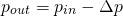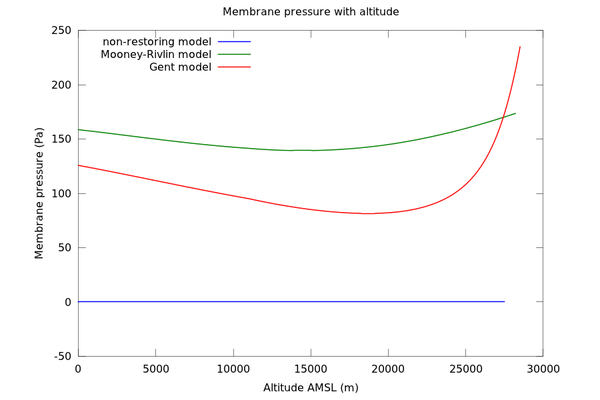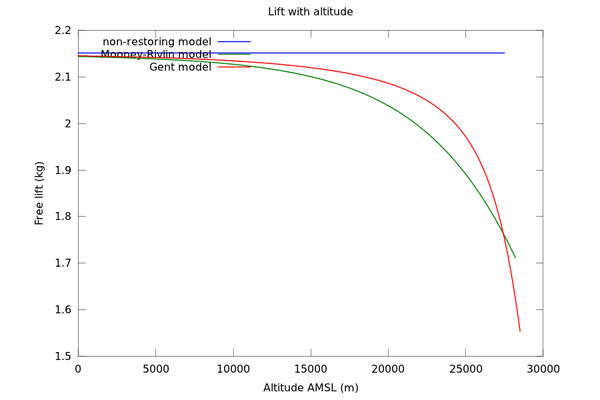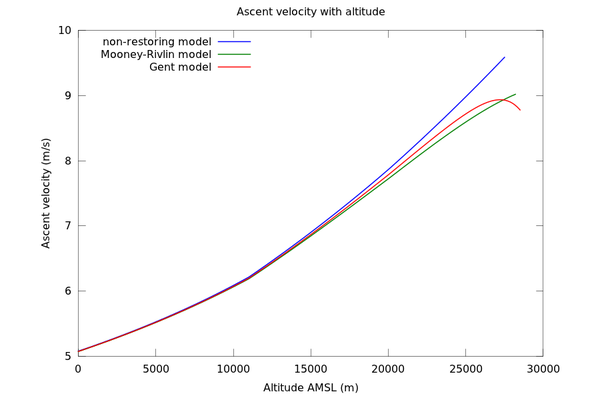# Weather balloon physics

One of the simplest solutions for sending measurement instruments up into the stratosphere is a rubber balloon filled with hydrogen or helium. While the physics of such a balloon would seem to be simple, there are actually some interesting considerations.

For any elastic balloon, the pressure inside is always a little greater than the pressure outside, since the rubber exerts a restoring force inwards. For maximum lift, we want to minimise the density of the gas inside the balloon, so this extra pressure is not desirable. My goal was to model how much of an effect this has on the lift produced.

I will work through an example using a Novalynx 400-8242 balloon. The reason I have chosen this balloon is that test data is readily available on the web page.

Let us write the pressure equation as:pout, the pressure outside the balloon, is a function of height and can be approximated from a standard atmospheric model. pin can be calculated from the gas law, as a function of the number of moles of gas in the balloon (a constant throughout the ascent), the volume of the balloon, and temperature. In order to calculate Δp, we need a stress-strain model of the balloon rubber [1,2]. Here we use two well-known hyperelasticity models: the Mooney-Rivlin model and the Gent model. The Gent model has the advantage of modelling the stiffening that occurs as the rubber approaches breaking point, but the parameters are more difficult to determine accurately without performing destructive balloon measurements.

For the Mooney-Rivlin model we obtain:We use the typical values given in  for the shear modulus μ and the parameter α (μ=300 kPa and α=10/11). In the Gent model, only the final term in parentheses is different; it includes a Jm parameter related to the maximum possible stretch.

r0 (the unstretched radius) for the balloon is given by the manufacturer. Using r0, the mass of the balloon, and the density of rubber, we can also approximate t0 (the unstretched thickness), which works out as approximately 0.2mm. (In the same way we can estimate the thickness at bursting to be approximately 5μm. Other sources  suggest a value of approximately 3.4μm for the balloons used in their experiments, which may be due to differences in the unstretched dimensions or in the manufacturing process.)

By setting r to the initial inflation radius at h=0, we can obtain n (the number of moles of gas in the balloon). Then, we can solve the above equation for r at each altitude h.

Figure 1 shows the resulting graph of balloon radius vs height. As well as the Mooney-Rivlin model and the Gent model, the graph also includes a purely theoretical non-restoring model, in which the balloon exerts no restoring force at all (Δp=0).The burst radius of the balloon is given by the manufacturer as 3.4m. This occurs at 27.5km for the non-restoring model, 28.2km for the Mooney-Rivlin model and 28.5km for the Gent model with the chosen parameters. All of these figures are close to the performance test data, which indicates a burst height of 28km. So it does not seem that elasticity has much of an effect on the ceiling in this particular example.

Figure 2 shows the pressure difference across the balloon membrane for each model:For the type of balloon at hand (with given t0/r0), it can be seen that the membrane pressure is of the order of 100-200 Pa throughout the flight envelope. This means that the difference in pressure between inside and outside is negligible at low altitudes (sea level pressure is of the order of 100,000 Pa). However, at high altitudes it may start to become important. At 28km the pressure has fallen to 1600 Pa; at 40km it is 290 Pa.

The significance of this becomes more evident when we plot the lift curve:In the non-restoring model with no pressure difference between inside and outside, constant lift is maintained. However, this is not true when elasticity is considered. In the case of the Gent model the free lift drops from 2.1kg to 1.5kg as the balloon approaches its burst point (the exact value has some uncertainty due to choice of Gent model parameters). In the present example this is not sufficient to arrest the ascent of the balloon, but it may be important to consider if the target altitude is higher and/or the balloon already has marginal lift performance.

Finally the ascent rate was calculated based on a 0.47 drag co-efficient for a sphere:Notice that the ascent rate increases with height — the reason is that air density (and hence drag) drops more sharply with height than lift does. The initial ascent rate is 5m/s, or 300m/min, which is lower than the tabulated 400m/min. However, the average ascent rate is almost exactly 400m/min, so it is likely that this is what is shown in the performance table. (The whole ascent to 28.5km takes 71.4 minutes.)

The Octave/MATLAB functions used to plot these graphs are available here.

References:
 Ingo Müller and Peter Strehlow. Rubber and rubber balloons: paradigms of thermodynamics. Lecture Notes in Physics, Springer, 2004
 Landon M. Kanner. Inflation of strain-stiffening rubber-like thin spherical shells. Proceedings of the Virginia Space Grant Consortium Student Research Conference, April 2007
 Noboyuki Yayima et al. Scientific ballooning: technology and applications of exploration balloons floating in the stratosphere and the atmospheres of other planets. Lecture Notes in Mathematics, Springer, 2009

This entry was posted in Physics. Bookmark the permalink.

### 10 Responses to Weather balloon physics

1.matt says:

What about lots of rubber gloves?

•Matthew says:

Ha. Someone suggested condoms. It’s a lot of extra rubber compared to a single sphere though — apart from the story factor, a balloon seems rather more fit-for-purpose.

•matt says:

Interesting to think about it in the context of the FAA 4kg requirement…

2.Manuel Cornejo Muñoz says:

Hi Matt

So it seems to me that you like to fly balloons, at least from a theoretical point of view, don’t you? Have you ever thought in the challenge of a “cuasipermanent staratospheric balloon” ? -55 ºC all the time affects the material. Maybe the antartic balloons use by NASA https://www.nasa.gov/content/goddard/nasa-funded-science-balloons-launch-in-antarctica/#.VVCiLeGXz3c, could be the answer to this challenge. Do you now if someone could buy one of this and where?

Sincerely

Manuel Cornejo

3.Marcriv says:

Why is 300m/min the desired ascent rate for weather balloons?